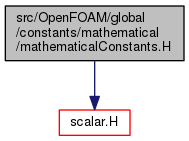The OpenFOAM Foundation
mathematicalConstants.H File Reference
Include dependency graph for mathematicalConstants.H:Go to the source code of this file.

## Namespaces

Foam::constant::mathematical
mathematical constants.

Foam
Namespace for OpenFOAM.

Foam::constant
Collection of constants.

## Functions

const scalar e (M_E)

const scalar pi (M_PI)

const scalar twoPi (2 *pi)

const scalar piByTwo (0.5 *pi)

const scalar Eu (0.57721566490153286060651209)
Euler's constant. More...

## Variables

static const char *const group = "mathematical"

## Detailed Description

Original source file mathematicalConstants.H

Definition in file mathematicalConstants.H.